1. Worksheets>
2. Math>
3. Geometry>
4. Angles>
5. Measuring Angles

Measuring Angles and Protractor Worksheets

This page contains measuring angles using a protractor, measuring angles formed by multiple rays, measuring angles formed between hands of the clocks, drawing minute hands, measuring angles in geometric shapes, reading protractor, drawing angles with a protractor, estimating angle measures, comparing estimation with exact measure, and simple variable solving problems.

Measuring Angle with Protractor: Type 1

Each worksheet has 8 problems measuring angles with a protractor. Easy level has an increment by 5s, and moderate level has an increment by 1s. For the difficult one, the angles spun any directions with the increment by 1s.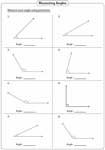Easy:

Moderate:

Difficult:

Measuring Angle with Protractor: Type 2

You have rays with sides and vertex marked. Find the angles using a protractor. See above to know details about difficulty levels.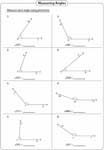Easy:

Moderate:

Difficult:

Multiple Rays

Measure the angles formed by multiple rays over a line having a common vertex.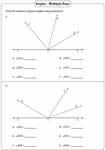Angle between Hands of a Clock

Using a protractor, find the measure of angle formed between hour hand and minute hand in each clock.Drawing Minute Hand

The angles between the hands are given. Keep the protractor at the hour hand and draw a minute hand for the given degree. Caution: It seems you have two choices, but there is only one.Angles in Shapes

Students should find only one angle in each geometric shape.Read the protractor and find the angle formed between a pair of lines in each question.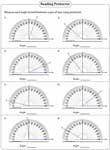Level 1:

Level 2:

Drawing Angles with Protractor

Each worksheet has six problems drawing angles using a protractor.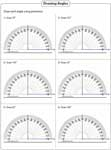Our Own Protractor:

Easy:

Moderate:

Easy:

Moderate:

Estimation vs Exact Measure

Estimate the measure of each angle. Calculate the exact measurement using a protractor. Compare the results.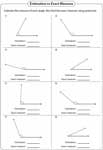Estimating Angles

Choose the best estimation for each angle. Moderate level includes reflex angle.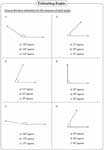Easy:

Moderate:

Finding Value of X

Each angle is given in algebraic expression. Set this expression equal to the given angle and solve for x.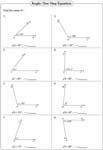One-step:

Two-step:

Stock up your library with the complete set of worksheets on measuring angles and protractor.

Related Worksheets